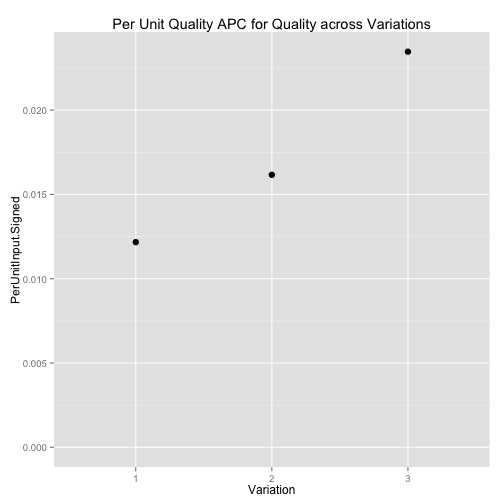# Predcomps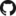Home

PDF Manual

Per Unit Input (original APC)

Impact (in units of output)

## Examples

Examples Overview

A fake logistic regression example predicting wine sales

A linear model with interactions

Loan defaults example

## More

As compared with Gelman & Pardoe 2007

Pairs & Weights

## A Logistic Regression with Related Inputs

(The source code for this example is here.)

We will set up a simulated data set to use for modeling the probability a customer buys a bottle of wine, given its price and quality. We'll compare a few situation varying the joint distribution of price ($$P$$) and quality ($$Q$$). The coefficients of the logistic regression determining the relationship between the inputs and the probability of purchase will not vary.

In each variation, the probability of purchase is governed by the following logistic regression model:

$logit(P(\text{wine is purchased})) = 0.1 Q - 0.12 P$

• Variation 1: Price is uniform; quality is price plus noise. Quality increases with price, but not enough make up for price, so the expensive wines are rarely purchased and the cheap wines are almost always purchased.
• Variation 2: Just like Variation 1, but price is more densely concentrated in its middle range. This leads to price and quality both having a larger APC because the inverse logistic curve is steeper in this middle range.
• Variation 3: Like Variation 1, but quality varies more strongly with price. The inverse logistic curve is now steeper at almost all price/quality combinations.

The APC varies across these variations, but the logistic regression coefficients remain the same. The changes in APC in each of these variations are driven entirely by changes in the distribution of the inputs. The model relating inputs to outputs is unchanged.

### Variation 1

In the first variation, quality and price are independent, with price uniformly distributed and quality set to price plus Gaussian noise:

priceCoef <- -.12
qualityCoef <- .1
qualityNoiseStdDev <- 5
nWines=50000
nRowsForPlottingSample <- 1000

numForTransitionStart <- 500
numForTransitionEnd <- 10000
onlyIncludeNearestN = 100

priceQualitySlope <- .4

df1 <- local({
price <- sample(20:120, nWines, replace=TRUE)
quality <- price * priceQualitySlope + 22 + rnorm(nWines, sd=qualityNoiseStdDev)
purchaseProbability <- inv.logit(priceCoef*(price - 70) + qualityCoef*(quality - 50)  )
purchased  <- rbinom(n = length(purchaseProbability), size=1, prob=purchaseProbability)
data.frame(Quality = quality,
Price = price,
PurchaseProbability = purchaseProbability,
Purchased = purchased)
})
print(getwd())

##  "/Users/david/github/predcomps/notes/examples"


A scatter plot (using a random subset to avoid overplotting) shows us the relationship between price and quality:

df1Sample <- df1[sample.int(nWines, size=nRowsForPlottingSample), ]
qplot(Price, Quality, alpha=I(.5), data = df1Sample) +
expand_limits(y=c(0,100))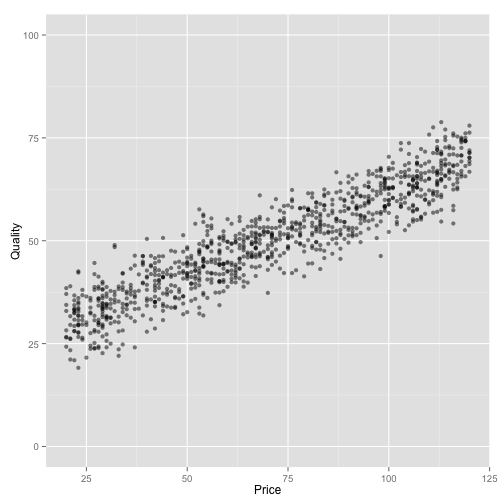When we fit a logistic regression, the coefficients are what we'd expect from the setup above:

logitFit1 <- glm(Purchased ~ Price + Quality, data = df1, family = "binomial")
logitFit1

##
## Call:  glm(formula = Purchased ~ Price + Quality, family = "binomial",
##     data = df1)
##
## Coefficients:
## (Intercept)        Price      Quality
##       3.357       -0.122        0.103
##
## Degrees of Freedom: 49999 Total (i.e. Null);  49997 Residual
## Null Deviance:       69300
## Residual Deviance: 37700     AIC: 37700


This plot shows the relationship between quality and probability of purchase for a few prices:

myScales <- list(scale_x_continuous(limits=c(0,100)),
scale_y_continuous(limits=c(0,1)))

ggplot(subset(df1Sample, Price %in% seq(20, 120, by=10))) +
geom_point(aes(x = Quality, y = PurchaseProbability, color = factor(Price)),
size = 3, alpha = 1) +
ggtitle("Quality vs. Purchase Probability at Various Prices") + myScales +
scale_color_discrete("Price")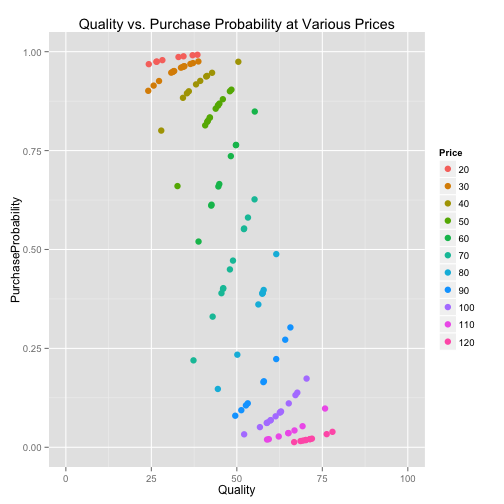Each colored set of points is one portion of a shifted inverse logistic curve, determined by which Price/Quality combinations actually occur in our data.

We can also see the portion of the curve that isn't represented in our data:

## Warning: Removed 550 rows containing missing values (geom_path).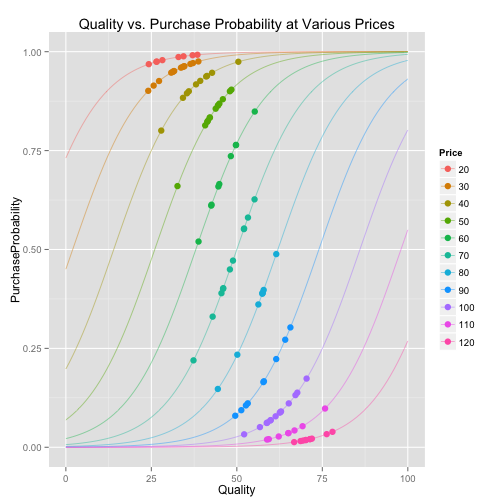We can get average predictive comparisons from our fitted regression:

apc1 <- GetPredCompsDF(logitFit1, df1,
numForTransitionStart = numForTransitionStart,
numForTransitionEnd = numForTransitionEnd,
onlyIncludeNearestN = onlyIncludeNearestN)

## Working on: Price
## Working on: Quality


The GetPredCompsDF function produces a few kinds of outputs, but for now let's just focus on the signed average predictive comparison:

apc1[c("Input", "PerUnitInput.Signed")]

##           Input PerUnitInput.Signed
## Price     Price            -0.01668
## Quality Quality             0.01217


This means that (on average) the probability of purchase increases by about 1.2% per 1 unit increase in quality.

### Variation 2

This variation will add some additional wines to the middle range of prices:

nAdditionalWines <- nWines
supplementForDF2 <- local({
price <- sample(55:85, nWines, replace=TRUE)
quality <- price * .4 + 22 + rnorm(nWines, sd=qualityNoiseStdDev)
purchaseProbability <- inv.logit(priceCoef*(price - 70) + qualityCoef*(quality - 50)  )
purchased  <- rbinom(n = length(purchaseProbability), size=1, prob=purchaseProbability)
data.frame(Quality = quality,
Price = price,
PurchaseProbability = purchaseProbability,
Purchased = purchased)
})
df2 <- rbind(df1, supplementForDF2)


A scatter plot (again, using a random subset to avoid overplotting) shows us the relationship between price and quality:

df2Sample <- df2[sample.int(nrow(df2), size=nRowsForPlottingSample), ]
qplot(Price, Quality, alpha=I(.5), data = df2Sample) +
expand_limits(y=c(0,100))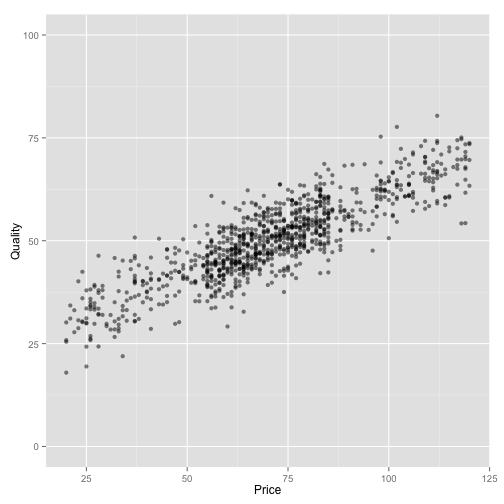When we fit a logistic regression, the coefficients are similar to before, since we haven't changed the underlying model:

logitFit2 <- glm(Purchased ~ Price + Quality, data = df2, family = "binomial")
logitFit2

##
## Call:  glm(formula = Purchased ~ Price + Quality, family = "binomial",
##     data = df2)
##
## Coefficients:
## (Intercept)        Price      Quality
##      3.4404      -0.1199       0.0991
##
## Degrees of Freedom: 99999 Total (i.e. Null);  99997 Residual
## Null Deviance:       139000
## Residual Deviance: 99300     AIC: 99300


In the plot showing the relationship between quality and probability of purchase, we see more points at the steep section of the inverse logit curve:

ggplot(subset(df2Sample, Price %in% seq(20, 120, by=10))) +
geom_point(aes(x = Quality, y = PurchaseProbability, color = factor(Price)),
size = 3, alpha = 1) +
ggtitle("Quality vs. Purchase Probability at Various Prices") +
myScales +
scale_color_discrete("Price")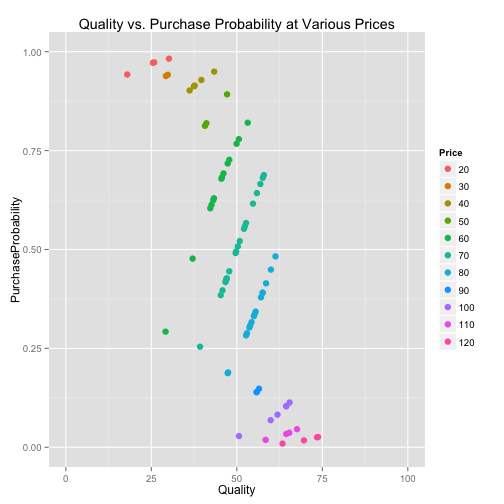The APC for quality is correspondingly larger:

apc2 <- GetPredCompsDF(logitFit2, df2,
numForTransitionStart = numForTransitionStart,
numForTransitionEnd = numForTransitionEnd,
onlyIncludeNearestN = onlyIncludeNearestN)

## Working on: Price
## Working on: Quality


apc2[c("Input",  "PerUnitInput.Signed")]

##           Input PerUnitInput.Signed
## Price     Price            -0.02019
## Quality Quality             0.01616


This means that in this variation the probability of purchase increases (on average) by about 1.5% (vs. 1.2% in Variation 1) per 1-point increase in quality. The magnitude of the APC for price is also larger.

### Variation 3

This is just like Variation 1, but price increases more with quality:

priceQualitySlope <- 1.2

df3 <- local({
price <- sample(20:120, nWines, replace=TRUE)
quality <- price * priceQualitySlope - 30 + rnorm(nWines, sd=qualityNoiseStdDev)
purchaseProbability <- inv.logit(priceCoef*(price - 70) + qualityCoef*(quality - 50)  )
purchased  <- rbinom(n = length(purchaseProbability), size=1, prob=purchaseProbability)
data.frame(Quality = quality,
Price = price,
PurchaseProbability = purchaseProbability,
Purchased = purchased)
})

df3Sample <- df3[sample.int(nWines, size=nRowsForPlottingSample), ]
qplot(Price, Quality, alpha=I(.5), data = df3Sample) +
expand_limits(y=c(0,100))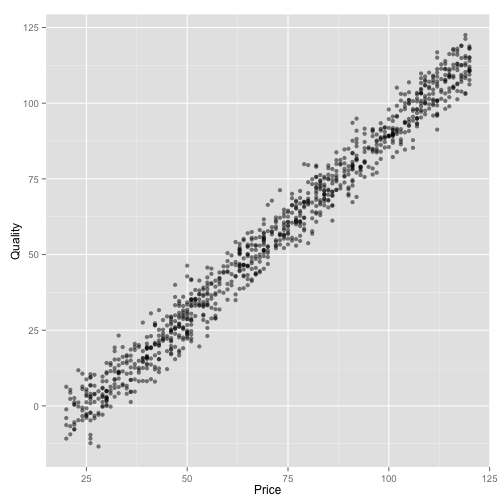The logistic regression still comes out the same:

logitFit3 <- glm(Purchased ~ Price + Quality, data = df3, family = "binomial")
logitFit3

##
## Call:  glm(formula = Purchased ~ Price + Quality, family = "binomial",
##     data = df3)
##
## Coefficients:
## (Intercept)        Price      Quality
##       3.465       -0.122        0.102
##
## Degrees of Freedom: 49999 Total (i.e. Null);  49997 Residual
## Null Deviance:       67700
## Residual Deviance: 64800     AIC: 64800


In this case, purchase is less certain at the low prices and more plausible at the high prices:

ggplot(subset(df3Sample, Price %in% seq(-100, 200, by=10))) +
geom_point(aes(x = Quality, y = PurchaseProbability, color = factor(Price)),
size = 3, alpha = 1) +
ggtitle("Quality vs. Purchase Probability at Various Prices") +
myScales +
scale_color_discrete("Price")

## Warning: Removed 25 rows containing missing values (geom_point).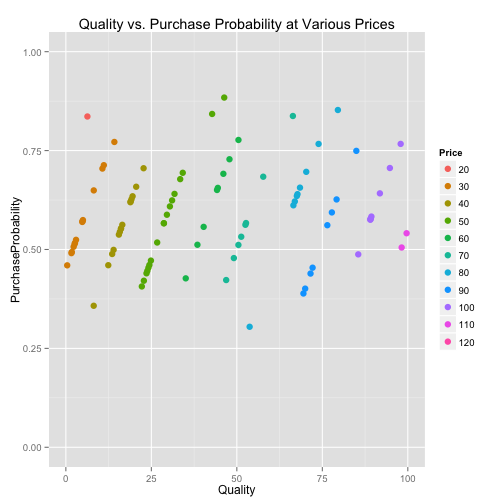We can get average predictive comparisons from our fitted regression:

apc3 <- GetPredCompsDF(logitFit3, df3,
numForTransitionStart = numForTransitionStart,
numForTransitionEnd = numForTransitionEnd,
onlyIncludeNearestN = onlyIncludeNearestN)

## Working on: Price
## Working on: Quality


As expected, the APCs are (both) larger than in Variation 1:

apc3[c("Input", "PerUnitInput.Signed")]

##           Input PerUnitInput.Signed
## Price     Price            -0.02809
## Quality Quality             0.02347


### Comparing the Variations

Comparing all of the variation in one plot, we can see the increase in the effect of wine quality on purchase probability going from Variation 1 to Variation 3: